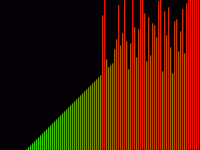# The Basic Principles of Quick Sort Algorithm

Written by Raza. Posted in C++We left our series of Sorting Algorithms about two months ago. Till then we discussed many in-place sorting algorithms like insertion sort, bubble sort, selection sort and gnome sort. These all were the basic algorithms which were inefficient for sorting large lists. For large amount of data, very advanced sorting algorithms have been developed by the experts and a lot of research is still going on in this area. According to many, the fastest Sorting Algorithm in Computer Science is the Quick Sort Algorithm. I shall explain this brilliant algorithm to you in this article in detail. Afterwards we would together write a source code for that.

# Selection Sort, the Basic Sorting Algorithm

Written by Raza. Posted in C++Selection sort is one of the basic sorting Algorithms. It is pretty simple to understand and very good to implement on small amount of data. However, it becomes quite inefficient when working with large lists.

Selection Sort uses the concept of in-place sorting. It means that the data is sorted by shuffling it within the list. First I shall try to explain the algorithm and then together we would try to carve out of it a basic source code.

# Gnome Sort, a Variant of Insertion Sort

Written by Raza. Posted in C++Last time, we discussed a sorting technique known as insertion sort. It is a very good sorting procedure and the simplest sorting algorithm for short lists and small amount of data. For larger lists, it’s running time increases exponentially which renders it inefficient. A number of variations have also been introduced to insertion sort, some of which are more efficient and some are equally good. Shell sort and Gnome Sort are two such variations. I prefer Gnome Sort so I will explain it to you in this article.